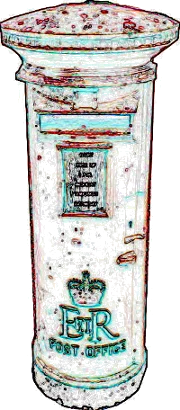## Print

### IntroductionIn principle, every computer program has to communicate with the environment or the "outside world". For this purpose nearly every programming language has special I/O functionalities, i.e. input/output. This ensures the interaction or communication with the other components e.g. a database or a user. Input often comes - as we have already seen - from the keyboard and the corresponding Python command, or better, the corresponding Python function for reading from the standard input is input().

We have also seen in previous examples of our tutorial that we can write into the standard output by using print. In this chapter of our tutorial we want to have a detailed look at the print function. As some might have skipped over it, we want to emphasize that we wrote "print function" and not "print statement". You can easily find out how crucial this difference is, if you take an arbitrary Python program written in version 2.x and if you try to let it run with a Python3 interpreter. In most cases you will receive error messages. One of the most frequently occurring errors will be related to print, because most programs contain prints. We can generate the most typical error in the interactive Python shell:

print 42
File "", line 1
print 42

  File "<ipython-input-1-5d54469f7ddf>", line 1
print 42
^
SyntaxError: Missing parentheses in call to 'print'. Did you mean print(42)?


This is a familiar error message for most of us: We have forgotten the parentheses. "print" is - as we have already mentioned - a function in version 3.x. Like any other function print expects its arguments to be surrounded by parentheses. So parentheses are an easy remedy for this error:

print(42)

42


But this is not the only difference to the old print. The output behaviour has changed as well.

The arguments of the print function are the following ones:

 print(value1, ..., sep=' ', end='\n', file=sys.stdout, flush=False)
The print function can print an arbitrary number of values ("value1, value2, ..."), which are separated by commas. These values are separated by a space in the output. In the following example, we can see two print calls. We are printing two values in both cases, i.e. a string and a float number:

a =  3.564
print("a = ", a)

a =  3.564

print("a = \n", a)

a =
3.564


We can learn from the second print of the example that a blank between two values, i.e. "a = \textbackslash n" and "3.564", is always printed, even if the output is continued in the following line. This is different from Python 2, as there will be no blank printed, if a new line has been started. It's possible to redefine the seperator between values by assigning an arbitrary string to the keyword parameter "sep", i.e. an empty string or a smiley:

print("a","b")

a b

print("a","b",sep="")

ab

print(192,168,178,42,sep=".")

192.168.178.42

print("a","b",sep=":-)")

a:-)b


A print call is ended by a newline, as we can see in the following usage:

for i in range(4):
print(i)

0
1
2
3


To change this behaviour, we can assign an arbitrary string to the keyword parameter "end". This string will be used for ending the output of the values of a print call:

for i in range(4):
print(i, end=" ")

0 1 2 3
for i in range(4):
print(i, end=" :-) ")

0 :-) 1 :-) 2 :-) 3 :-)

The output of the print function is send to the standard output stream (sys.stdout) by default. By redefining the keyword parameter "file" we can send the output into a different stream e.g. sys.stderr or a file:

fh = open("data.txt","w")
print("42 is the answer, but what is the question?", file=fh)
fh.close()


We can see that we don't get any output in the interactive shell. The output is sent to the file "data.txt". It's also possible to redirect the output to the standard error channel this way:

import sys
# output into sys.stderr:

print("Error: 42", file=sys.stderr)

Error: 42